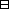## Group Theory and Linear Algebra

Last updated: 17 September 2014

## Lecture 3: Equivalence relations

A set is a collection of elements.

Let $S$ and $T$ be sets. The product of $S$ and $T$ is the set $S×T= { (s,t) | s∈S,t∈T } .$

If $S=\left\{1,2,3\right\}$ then $S×S= { (1,1), (1,2), (1,3) (2,1), (2,2), (2,3) (3,1), (3,2), (3,3) } .$

Let $S$ be a set. A relation on $S$ is a subset of $S×S\text{.}$

$<$ is a relation on $ℤ\text{.}$ $a if there exists $x\in {ℤ}_{>0}$ such that $a+x=b\text{.}$ $a means $\left(a,b\right)$ is in the relation $<\text{.}$

Let $m\in ℤ\text{.}$ Let $a,b\in ℤ\text{.}$ Define $\phantom{\rule{1em}{0ex}}=\phantom{\rule{1em}{0ex}}\text{mod} m,$ a relation on $ℤ,$ by $a=b mod mif ra=rb,$ where $a={q}_{a}m+{r}_{a}$ and $b={q}_{b}m+{r}_{b}$ with ${q}_{a},{q}_{b}\in ℤ$ and $0\le {r}_{a}<|m|$ and $0\le {r}_{b}<|m|\text{.}$

Define $a \text{mod} m$ to be ${r}_{a},$ where $a={q}_{a}m+{r}_{a}$ with ${q}_{a}\in ℤ$ and $0\le {r}_{a}<|m|\text{.}$

Let $S$ be a set. Let $\sim$ be a relation on $S\text{.}$ Write ${s}_{1}\sim {s}_{2}$ if $\left({s}_{1},{s}_{2}\right)$ is in the relation $\sim \text{.}$

The relation $\sim$ is reflexive if $\sim$ satisfies: if $s\in S$ then $s\sim s\text{.}$

The relation $\sim$ is symmetric if $\sim$ satisfies: if ${s}_{1},{s}_{2}\in S$ and ${s}_{1}\sim {s}_{2}$ then ${s}_{2}\sim {s}_{1}\text{.}$

The relation $\sim$ is transitive if $\sim$ satisfies: if ${s}_{1},{s}_{2},{s}_{3}\in S$ and ${s}_{1}\sim {s}_{2}$ and ${s}_{2}\sim {s}_{3}$ then ${s}_{1}\sim {s}_{3}\text{.}$

An equivalence relation on $S$ is a relation on $S$ that is reflexive, symmetric and transitive.

Let $S$ be a set. Let $\sim$ be an equivalence relation on $S\text{.}$ Let $s\in S\text{.}$ The equivalence class of $s$ is the set $[s]= {x∈S | x∼s}.$

A partition of $S$ is a collection $𝒮$ of subsets of $S$ such that

 (a) $\bigcup _{Y\in 𝒮}Y=S\text{.}$ (b) If $X,Y\in 𝒮$ and $X\ne Y$ then $X\cap Y=\varnothing \text{.}$

Let $m=7\text{.}$ Then $36 mod 7 = 1,since 36=5·7+1, -6 mod 7 = 1, since-6=-1·7+1, 1 mod 7 = 1,since 1=0·7+1.$ The equivalence class of $36$ is $= { …,-13,-6,1,8,15 ,22,29,36,… } = , { …,-12,-5,2,9, 16,23,30,37,… } = , { …,-11,-4,3,10 ,17,24,… } = , { …,-10,-3,11, 18,25,… } = , { …,-9,-2,5,12, 19,26,… } = , { …,-8,-1,6,13, 20,27,… } = , { …,-7,0,7,14,21 ,28,… } = .$ Recall that $ℤ}{mℤ}=\left\{1,2,3,4,5,6,7\right\}\text{.}$

Note that ${ , , , , , ,  }$ is a partition of $ℤ$ since

 (a) $\left[1\right]\cup \left[2\right]\cup \left[3\right]\cup \left[4\right]\cup \left[5\right]\cup \left[6\right]\cup \left[7\right]$ and (b) if $i,j\in \left\{1,\dots ,7\right\}$ and $i\ne j$ then $[i]∩[j]=∅.$

Let $m\in ℤ\text{.}$ Then $= \text{mod} m$ is an equivalence relation on $ℤ\text{.}$Proof.

To show:
 (a) $= \text{mod} m$ is reflexive. (b) $= \text{mod} m$ is symmetric. (c) $= \text{mod} m$ is transitive.
To show:
 (a) If $a\in ℤ$ then $a=a \text{mod} m\text{.}$ (b) If $a,b\in ℤ$ and $a=b \text{mod} m$ then $b=a \text{mod} m\text{.}$ (c) If $a,b,c\in ℤ$ and $a=b \text{mod} m$ and $b=c \text{mod} m$ then $a=c \text{mod} m\text{.}$
Assume $a,b,c\in ℤ$ and $a=b \text{mod} m$ and $b=c \text{mod} m\text{.}$
Let $a={q}_{a}·m+{r}_{a},$ $b={q}_{b}·m+{r}_{b},$ $c={q}_{c}·m+{r}_{c}$ with $0\le {r}_{a} $0\le {r}_{b} $0\le {r}_{c}
Since $a=b \text{mod} m$ and $b=c \text{mod} m$ then $ra=rband rb=rc.$ Since $=$ is an equivalence relation on $ℤ,$ $ra=ra, rb=raand ra=rc.$ So $a=a \text{mod} m,$ $b=a \text{mod} m$ and $a=c \text{mod} m\text{.}$
So $= \text{mod} m$ is an equivalence relation on $ℤ\text{.}$

$\square$

## Notes and References

These are a typed copy of Lecture 3 from a series of handwritten lecture notes for the class Group Theory and Linear Algebra given on July 29, 2011.